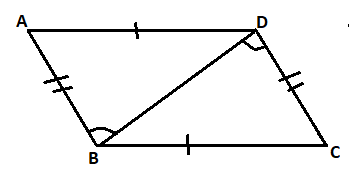QUESTION

# In the quadrilateral ABCD, AB = DC and AD = BC, then the sides AB and DC are parallel to each other.If the above statement is true, mention the answer as 1, else mention 0 if false.

Hint: Here we have to draw a quadrilateral and then prove that the triangle ABD and the triangle CBD are congruent to each other. After that using the CPCT rule (Corresponding parts of congruent triangles) we can prove that alternate interior angles are equal. And this will prove sides as parallel to each other.As we can know that sides AB and D are equal and sides AD and BC are also equal.
Let us take triangle ABD and CBD and check whether they are congruent to each other or not.
So, in the triangle $\Delta ABD$ and $\Delta CBD$.
AB = DC (given)
So, $\Delta ABD \cong \Delta CBD$ (By SSS congruence rule)
So, in triangle $\Delta ABD$ and $\Delta CBD$
We can say that $\angle ABD = \angle BDC$(CPCT Rule).
Now as we can see that $\angle ABD$ and $\angle BDC$ are the alternate interior angles. And we know that if two alternate interior angles of two sides are equal then the side must be parallel to each other.